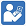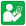Test Sheets - Divide by 1-Digit Numbers
Divide by 1-Digit Numbers - Test 1
Divide by 1-Digit Numbers - Test 2
Divide by 1-Digit Numbers - Test 3Practice Sheets - Divide by 1-Digit Numbers